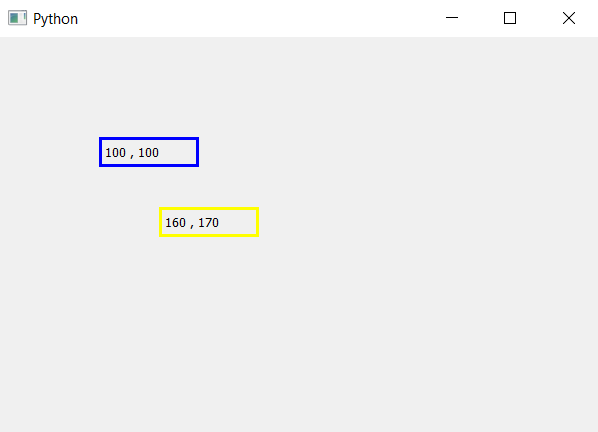# PyQt5 – How to get Label coordinates ?

• Last Updated : 26 Mar, 2020

The main window in PyQt5 is like graph it has x-axis and y-axis, all the widgets are positioned according to their x, y co-ordinates. When we create label is formed at the top left corner, the op left co-ordinates are (0, 0), we can move the label using `move()` method.
In this article, we will see how to get the co-ordinates of label. In order to do so we will use `x()` method for x co-ordinate and `y()` method for y co-ordinate.

Syntax :

```x_position = label.x()
y_position =label.y()
```

Argument : Both method takes no argument.

Return : Both returns integer.

Code :

 `# importing the required libraries ` ` `  `from` `PyQt5.QtCore ``import` `*`  `from` `PyQt5.QtGui ``import` `*`  `from` `PyQt5.QtWidgets ``import` `*`  `import` `sys ` ` `  ` `  `class` `Window(QMainWindow): ` ` `  ` `  `    ``def` `__init__(``self``): ` `        ``super``().__init__() ` ` `  ` `  `        ``# set the title ` `        ``self``.setWindowTitle(``"Python"``) ` ` `  `        ``# setting  the geometry of window ` `        ``self``.setGeometry(``60``, ``60``, ``600``, ``400``) ` ` `  ` `  `        ``# creating a label widget ` `        ``self``.label_1 ``=` `QLabel(``self``) ` ` `  `        ``# moving position ` `        ``self``.label_1.move(``100``, ``100``) ` ` `  `        ``# setting up the border ` `        ``self``.label_1.setStyleSheet(``"border :3px solid blue;"``) ` ` `  `        ``# getting x and y co-ordinates ` `        ``x ``=` `str``(``self``.label_1.x()) ` `        ``y ``=` `str``(``self``.label_1.y()) ` ` `  `        ``# setting label text ` `        ``self``.label_1.setText(x``+``", "``+` `y) ` ` `  `        ``# creating a label widget ` `        ``self``.label_2 ``=` `QLabel(``self``) ` ` `  `        ``# moving position ` `        ``self``.label_2.move(``160``, ``170``) ` ` `  `        ``# setting up the border ` `        ``self``.label_2.setStyleSheet(``"border :3px solid yellow;"``) ` ` `  `        ``# getting x and y co-ordinates ` `        ``x ``=` `str``(``self``.label_2.x()) ` `        ``y ``=` `str``(``self``.label_2.y()) ` ` `  `        ``# setting label text ` `        ``self``.label_2.setText(x ``+` `", "` `+` `y) ` ` `  `        ``# show all the widgets ` `        ``self``.show() ` ` `  ` `  `# create pyqt5 app ` `App ``=` `QApplication(sys.argv) ` ` `  `# create the instance of our Window ` `window ``=` `Window() ` `# start the app ` `sys.exit(App.``exec``()) `

Output :My Personal Notes arrow_drop_up
Recommended Articles
Page :